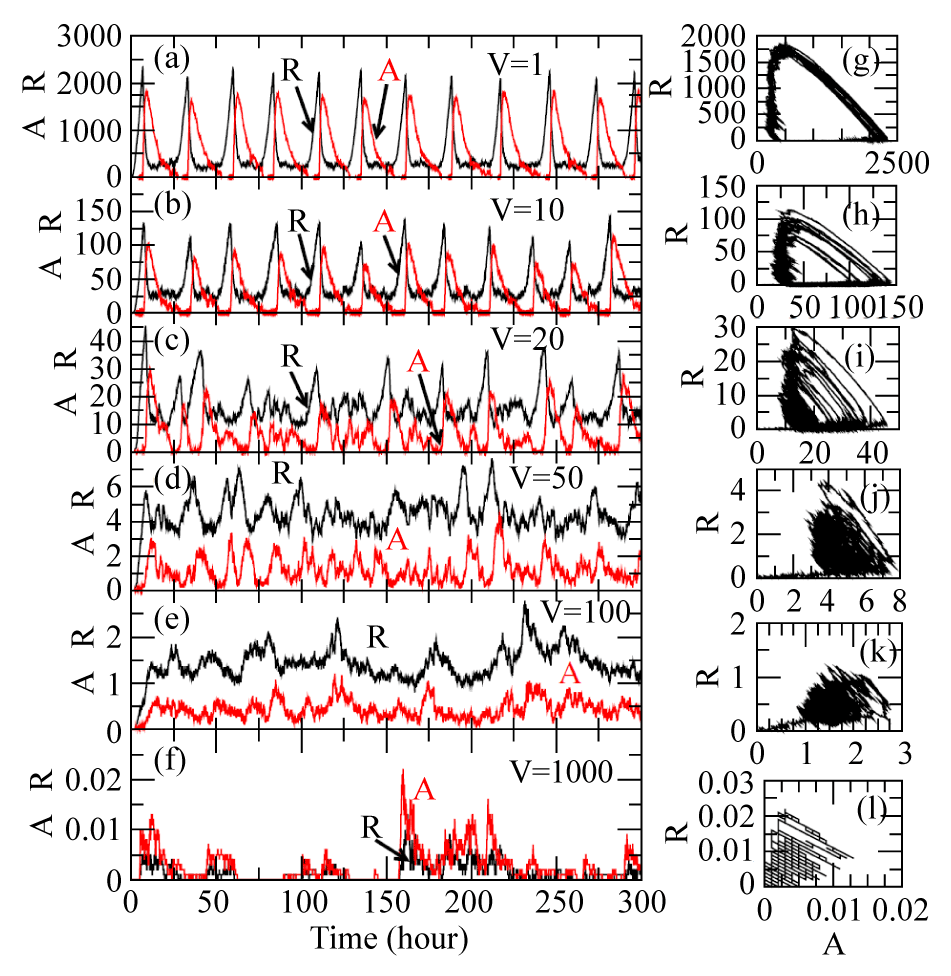Figure 1: The plots of the dynamics of protein concentrations A and R for different values of system size, V=1, 20, 50, 100 and 1000 respectively. The parameter values are taken from Vilar et al.  for fixed point oscillation. Panels (a), (b), (c), (d), (e) and (f) show the variations of concentrations of A and R as a function of time in hours for various values of V. Panels (g), (h), (i), (j), (k) and (l) are two dimensional plots of A as a function of R for corresponding values of V.